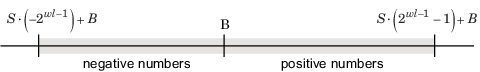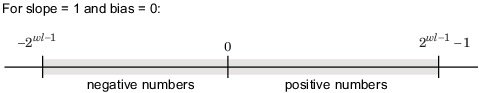Main Content

## Range and Precision

The range of a number gives the limits of the representation, while the precision gives the distance between successive numbers in the representation. The range and precision of a fixed-point number depend on the length of the word and the scaling.

Note

You must pay attention to the precision and range of the fixed-point data types and scalings you choose in order to know whether rounding methods will be invoked or if overflows or underflows will occur.

### Range

The range is the span of numbers that a fixed-point data type and scaling can represent. Range is limited because fixed-point words have limited size.

The range of representable numbers for a two's complement fixed-point number of word length $wl$, scaling $S$ and bias $B$ is illustrated below, where the values of $wl$, $S$, and $B$ allow for both negative and positive numbers.For both signed and unsigned fixed-point numbers of any data type, the number of different bit patterns is 2wl.

For example, in two's complement, negative numbers must be represented as well as zero, so the maximum value is 2wl -1 – 1. Because there is only one representation for zero, there are an unequal number of positive and negative numbers. This means there is a representation for $-{2}^{wl-1}$ but not for ${2}^{wl-1}$:#### Limitations on Range

Because a fixed-point data type represents numbers within a finite range, overflows and underflows can occur if the result of an operation is larger or smaller than the numbers in that range.

In binary arithmetic, a processor might need to take an n-bit fixed-point number and store it in m bits, where $m\ne n$. If m < n, the range of the number has been reduced and an operation can produce an overflow condition. Some processors identify this condition as `Inf` or `NaN`. For other processors, especially digital signal processors (DSPs), the value saturates or wraps.

Fixed-Point Designer™ software allows you to either saturate or wrap overflows. Saturation represents positive overflows as the largest positive number in the range being used, and negative overflows as the largest negative number in the range being used. Wrapping uses modulo arithmetic to cast an overflow back into the representable range of the data type.

When you create a `fi` object, any overflows are saturated. The `OverflowAction` property of the default fimath is `saturate`. You can log overflows and underflows by setting the `LoggingMode` property of the `fipref` object to `on`.

If m > n, the range of the number has been extended. Extending the range of a word requires the inclusion of guard bits, which act to guard against potential overflow.

The Simulink® software supports saturation and wrapping for all fixed-point data types, while guard bits are supported only for fractional data types.

### Precision

The precision of a fixed-point number is the difference between successive values representable by its data type and scaling. The value of the least significant bit, and therefore the precision of the number, is determined by the number of fractional bits. A fixed-point value can be represented to within half of the precision of its data type and scaling.

For example, a fixed-point representation with four bits to the right of the binary point has a precision of 2-4 or 0.0625, which is the value of its least significant bit. Any number within the range of this data type and scaling can be represented to within (2-4)/2 or 0.03125, which is half the precision. This is an example of representing a number with finite precision.

#### Limitations on Precision

The precision of a fixed-point word depends on the word size and binary point location. For example, suppose you must represent the real-world number 35.375 with a fixed-point number. Using a slope bias encoding scheme, the representation is

`$V\approx \stackrel{˜}{V}=SQ+B={2}^{-2}Q+32,$`

where V = 35.375.

The two closest approximations to the real-world value are Q = 13 and Q = 14:

`$\begin{array}{l}\stackrel{˜}{V}={2}^{-2}\left(13\right)+32=35.25,\\ \stackrel{˜}{V}={2}^{-2}\left(14\right)+32=35.50.\end{array}$`

In either case, the absolute error is the same:

`$|\stackrel{˜}{V}-V|=0.125=\frac{S}{2}=\frac{F{2}^{E}}{2}.$`

For fixed-point values within the limited range, this represents the worst-case error if round-to-nearest is used. If other rounding modes are used, the worst-case error can be twice as large:

`$|\stackrel{˜}{V}-V|`

Extending the precision of a word can be accomplished with more bits, but you face practical limitations with this approach. Instead, you must carefully select the data type, word size, and scaling such that numbers are accurately represented. Rounding and padding with trailing zeros are typical methods implemented on processors to deal with the precision of binary words.

### Fixed-Point Data Type Parameters

The low limit, high limit, and default binary-point-only scaling for the supported fixed-point data types discussed in Binary-Point-Only Scaling are given in the following table.

Fixed-Point Data Type Range and Default Scaling

Name

Data Type

Low Limit

High Limit

Default Scaling (~Precision)

Unsigned Integer

`fixdt(0,ws,0)`

0

${2}^{ws}-1$

`1`

Signed Integer

`fixdt(1,ws,0)`

$-{2}^{ws-1}$

${2}^{ws-1}-1$

`1`

Unsigned Binary Point

`fixdt(0,ws,fl)`

0

$\left({2}^{ws}-1\right){2}^{-fl}$

${2}^{-fl}$

Signed Binary Point

`fixdt(1,ws,fl)`

$-{2}^{ws-1-fl}$

$\left({2}^{ws-1}-1\right){2}^{-fl}$

${2}^{-fl}$

Unsigned Slope Bias

`fixdt(0,ws,s,b)`

`b`

$s\left({2}^{ws}-1\right)+b$

s

Signed Slope Bias

`fixdt(1,ws,s,b)`

$-s\left({2}^{ws-1}\right)+b$

$s\left({2}^{ws-1}-1\right)+b$

s

s = Slope, b = Bias, ws = WordLength, fl = FractionLength

### Range and Precision of an 8-Bit Fixed-Point Data Type — Binary-Point-Only Scaling

The precisions, range of signed values, and range of unsigned values for an 8-bit generalized fixed-point data type with binary-point-only scaling are listed in the follow table. Note that the first scaling value (21) represents a binary point that is not contiguous with the word.

Scaling

Precision

Range of Signed Values (Low, High)

Range of Unsigned Values (Low, High)

21

2.0

-256, 254

0, 510

20

1.0

-128, 127

0, 255

2-1

0.5

-64, 63.5

0, 127.5

2-2

0.25

-32, 31.75

0, 63.75

2-3

0.125

-16, 15.875

0, 31.875

2-4

0.0625

-8, 7.9375

0, 15.9375

2-5

0.03125

-4, 3.96875

0, 7.96875

2-6

0.015625

-2, 1.984375

0, 3.984375

2-7

0.0078125

-1, 0.9921875

0, 1.9921875

2-8

0.00390625

-0.5, 0.49609375

0, 0.99609375

### Range and Precision of an 8-Bit Fixed-Point Data Type — Slope and Bias Scaling

The precision and ranges of signed and unsigned values for an 8-bit fixed-point data type using slope and bias scaling are listed in the following table. The slope starts at a value of `1.25` with a bias of `1.0` for all slopes. Note that the slope is the same as the precision.

Bias

Slope/Precision

Range of Signed Values (low, high)

Range of Unsigned Values (low, high)

1

1.25

-159, 159.75

1, 319.75

1

0.625

-79, 80.375

1, 160.375

1

0.3125

-39, 40.6875

1, 80.6875

1

0.15625

-19, 20.84375

1, 40.84375

1

0.078125

-9, 10.921875

1, 20.921875

1

0.0390625

-4, 5.9609375

1, 10.9609375

1

0.01953125

-1.5, 3.48046875

1, 5.98046875

1

0.009765625

-0.25, 2.240234375

1, 3.490234375

1

0.0048828125

0.375, 1.6201171875

1, 2.2451171875

## SupportGet trial now